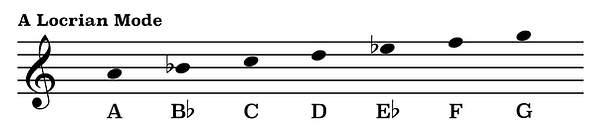top of page

## Mode Construction | Locrian Mode (7 of 7)

The notes of the Locrian mode are very similar to the notes of a natural minor scale. Like the Phrygian mode, the second scale degree of the Locrian mode is flattened by one half step. However, what makes the Locrian mode unique is the fact that it also contains a flattened fifth scale degree.

For this reason, the Locrian mode is the only scale discussed in this video series which will produce a diminished triad.

Here are the notes of an A minor scale.By flattening the second scale degree, B, as well as the fifth scale degree, E, we will be have constructed the A Locrian mode.Here now are the notes of a D natural minor scale.Again, by flattening the second scale degree, E, and the fifth scale scale degree, A, we will have now created the D Locrian mode.At the end of the previous video we briefly mentioned that the Locrian mode can be built on the seventh scale degree of a Major scale.

Looking at the notes of a C Major scale we can see that the seventh scale degree, or the seventh note in the scale, is the note B natural.Therefore, if we played all of the notes in the key of C Major from B natural to the next octave above we would then be playing the notes of B Locrian.Likewise, the seventh scale degree of a G Major scale is the note F-sharp.By playing all of the notes in the key of G Major from the note F-sharp to the next F-sharp one octave above we would then be playing F-sharp Locrian.Because the Locrian mode can be built off the seventh scale degree of a Major scale, the pattern of steps from which it is constructed is half step, whole step, whole step, half step, whole step, whole step, whole step.Let's use this pattern of steps to double check the notes of the B Locrian scale that we learned just a bit earlier.A half step above the note B is the note C. One half step above the note C is the note D. Another half step above D is the note E. A half step separates the notes E and F. The notes F and G are separated by a whole step. Another whole step separates the notes G and A. And lastly, a whole above the note A is the note B.

This pattern of steps may be used to construct a Locrian scale above any note.

In the beginning of this video we mentioned that one of the characteristics of the Locrian mode which makes it unique from the other modes discussed in this series is the flattened fifth scale degree. Seeing how a triad is constructed from the first, third, and fifth scale degrees of a corresponding scale, because of the flattened fifth scale degree a triad build from the notes of the Locrian mode will always be a diminished triad.

For example, we just constructed the notes of a B Locrian mode. We can see here that the first scale degree is the note B, the third scale degree is the note D, and the fifth scale degree is the note F.B, D, and F are in fact the notes of a B diminished triad.bottom of page